Next: 2. Modeling of data Up: 1.5 Learning of sensorimotor Previous: 1.5.5 Self-organizing maps

## 1.5.6 Parametrized self-organizing maps

Ritter (1993) presented an extension to the above SOM approach for learning sensorimotor maps, the `parametrized self-organizing map' (PSOM). The PSOM can cope with kinematic models of redundant robot arms (Walter et al., 2000). I first describe the training phase and then the recall phase.

The PSOM assumes that the training patterns lie on a sensorimotor manifold. In training, a continuous mapping is constructed that maps a parameter space (resembling the array in the SOM) onto the sensorimotor manifold. As for the SOM, the parameter space is based on an array of nodes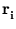, and each node i has a weight vector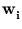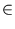IRd (here, element in the sensorimotor space). A continuous mapping from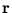to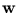is achieved by a sum of basis functions Hi() (one for each node i),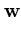(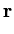) =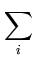Hi()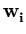. (1.6)

These basis functions are predefined and fulfill Hi(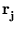) =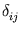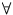i, j (Ritter, 1993). Thus, the mapping interpolates between the given grid points. Different from the SOM extension in section 1.5.5, only a few grid points are needed to describe a non-linear sensorimotor manifold. The grids usually have a side length of three points (Walter et al., 2000). In applications, mostly the motor components (for example, the joint angles of a robot arm) are chosen to form the parameter space, which further needs to be equidistantly sampled (Walter et al., 2000). The corresponding sensor values can be gained by executing the motor commands (or by taking the posture given by the joint angles).

The recall works like a pattern completion. The completion of a vector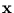is gained by finding the parameterthat minimizes the distance betweenand the sensorimotor manifold(). Sinceis only partially given, only the distance to the input components is evaluated,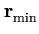= arg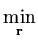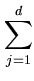gj(xj - wj())2 . (1.7)

Here, gj > 0 for input components, and gj = 0 for output components. The minimization needs to be solved numerically (Ritter, 1993; Walter et al., 2000).

This recall method has the advantage that it works in any direction. Thus, for example, a forward model can be changed into an inverse model by adjusting the gj values. Further, the method can be applied to redundant robot arms. Out of many possible postures, the one with the smallest distance to the manifold() is chosen, and not an average as in section 1.5.5.

The PSOM algorithm can achieve a remarkable accuracy (for example, a mean deviation of 1% of the working space for a three degrees-of-freedom robot finger (Walter et al., 2000)), but also here limitations exist. First, we need to know the topology of the sensorimotor patterns. Second, the mapping() requires a smooth sensorimotor manifold. Third, as in the previous approach, it is not clear how the algorithm can cope with noise dimensions.Next: 2. Modeling of data Up: 1.5 Learning of sensorimotor Previous: 1.5.5 Self-organizing maps
Heiko Hoffmann
2005-03-22